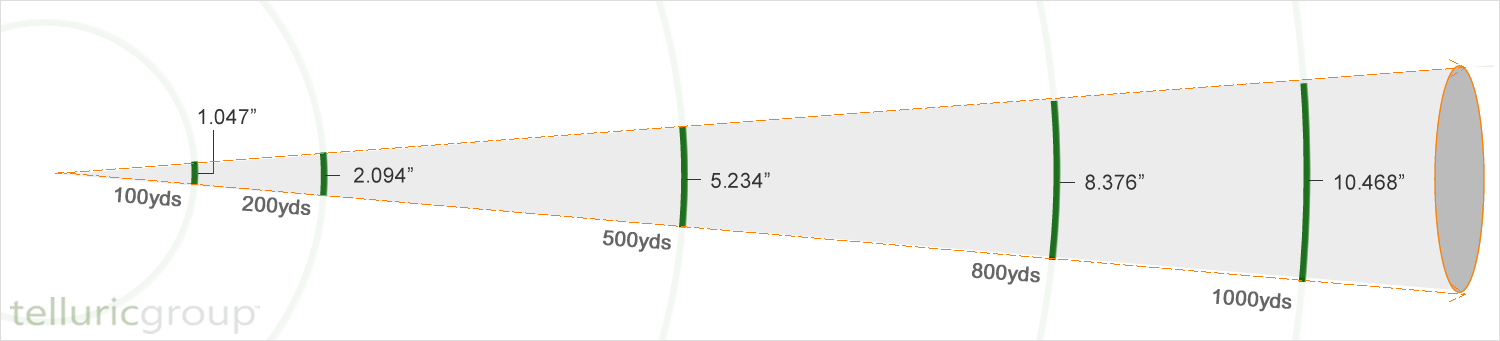# MINUTE OF ANGLE

‘Minute of Angle’ or ‘MOA’ is a term that’s used frequently to describe the accuracy of firearms, sights, ammunition, and other related equipment (i.e. ‘accurate to better than 1 MOA’). It’s also used as the basis for windage and elevation adjustments on quality sights and optics (i.e. ¼ MOA adjustments). Snipers become intimately familiar with the MOA during their training. It’s an important concept, though, for every rifleman- not just snipers- to thoroughly understand.# MOA DEFINED

The minute of angle (MOA) is a unit of angular measurement equal to 1/60th of 1 degree. There are 360 degrees in a circle (think about a compass dial). When more precise measurements are needed, each of those degrees can be divided into 60 minutes of angle (also called minutes of arc). So there are 60 MOA per degree, and a total of 21,600 MOA in a circle (360°x60’).

# MOA AND DISTANCE

Minute of angle (or degrees, or seconds of angle) can be thought of as a cone extending out from a point of origin ‘o’ (i.e. the rifle). As the distance (‘x’) between ‘o’ and the point of impact (‘i’) increases, the diameter (‘d’) of the base of the cone (the impact area for our rounds) also increases. How much the size of the impact area increases with distance depends on the size of the angle (‘a’) - larger angles yield larger impact areas. Diameter doubles, for instance, when the angle increases from 1MOA to 2MOA.

# SHOOTER'S MOA

For shooting, MOA allows us to:

1. predict the dispersion of bullet impacts at specified distances; and
2. calculate the shift in impact at a specified distance as a result of changes or adjustments made to the weapon or (more commonly) the sight.

Doing math under the time constraints and stress of combat (or even hunting), though, can be difficult. That's part of the reason that MOA is often simplified for shooting.   This simplified version is sometimes referred to as Shooters’ MOA (sMOA). For 1 sMOA:

diameter of impact area (inches) = range in yards / 100

So, if our range is 200 yards, a 1MOA impact area in inches is equal to 200 / 100, or 2 inches. If range is 25 yards, the impact area is 25 / 100, or 0.25 inches.